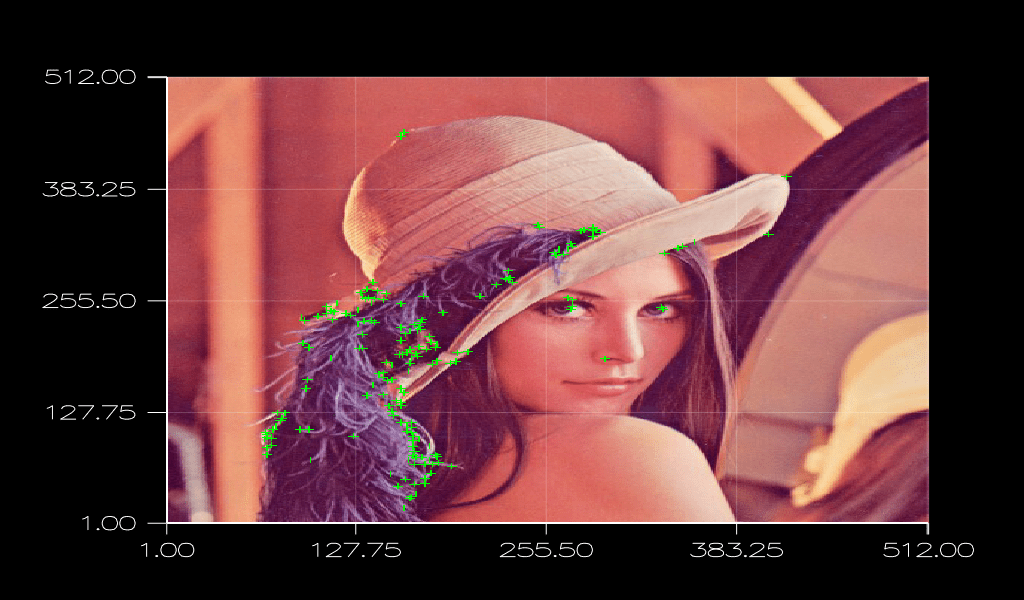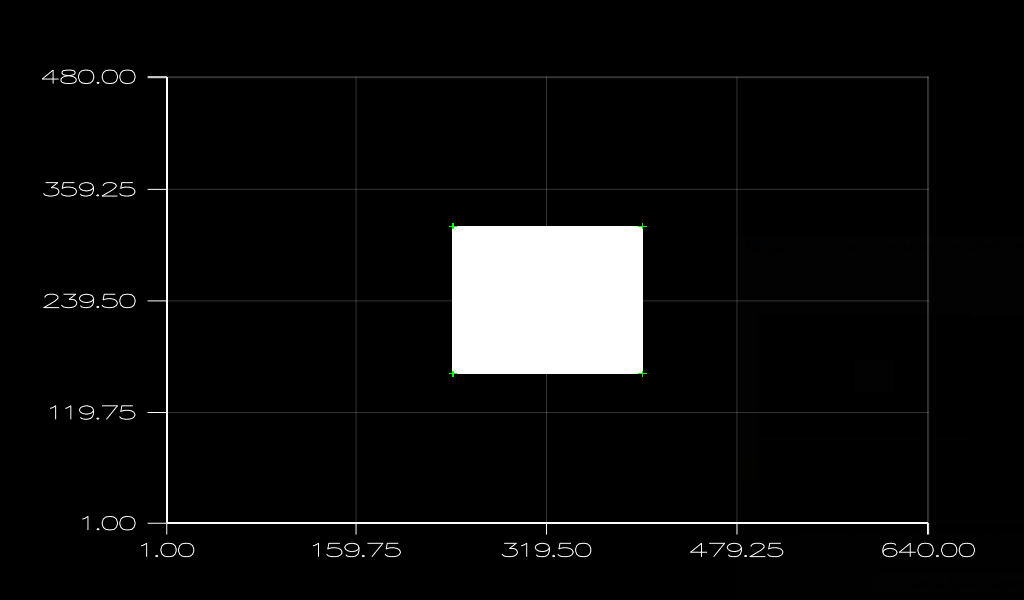# Writing a Simple Corner Detector with ArrayFire

In the upcoming months we’ll be adding a lot of new Computer Vision functionality to ArrayFire, specifically targeting the most commonly used applications in this field. New functions include feature tracking, object classification, scene segmentation, optical flow, and stereo-vision.

Feature tracking consists of three basic steps:

1. Detecting good or unique features; normally they are corners or blobs of an object.
2. Extracting a descriptor for each feature—understanding the texture of a small patch around each feature.
3. Descriptor matching—finding out the best match for each pair of descriptors (one from the object being tracked, another from a scene that potentially contains that object), if any.

### Harris corner detector

In this article we will be using ArrayFire to dive deeper into the first step of feature tracking: detecting good features. Through a step-by-step guide we will develop a simple corner detector, known as the Harris corner detector .

The Harris corner detector is very simple to implement, especially when you have so many good tools and libraries out there to do the core matrix manipulation. What we specifically need for Harris are some convolutions, together with a few element-wise matrix addition and multiplication operations; all of these operations are present in most scientific tools and libraries that work with matrix manipulation. I will try to avoid going into the mathematical details in this post and go directly to the implementation.

Before writing the code for the corner detector, we need to load an image. The image can be either color or grayscale, but it will only work on the grayscale version; if we upload a color image, we have to convert it before proceeding.

```// Load a color image
// Convert the image from RGB to gray-scale
array img_gray = colorspace(img_color, af_gray, af_rgb);
// For visualization in ArrayFire, color images must be in the [0.0f-1.0f] interval
img_color /= 255.f;
```
##### Compute first-order derivatives

The first step in the Harris corner detector is to determine first order derivatives of an image, in both horizontal and vertical directions. This can be achieved by one convolution for each direction, with the kernel (-1, 0, 1)  for horizontal derivative and its transpose for vertical derivative. ArrayFire contains a function called grad, which calculates gradients in both directions and will perform faster than the two convolutions. The only difference  is that grad divides the result by 2, thus, the response factor will change. The use of grad will improve the performance, and factor change can be easily compensated by using a smaller response.

```// Calculate image gradients
array ix, iy;
```
##### Compute second-order derivatives

At this point we have both horizontal and vertical first order derivatives of our image, but Harris corner detector is processed with second-order derivatives, which can be easily computed in ArrayFire with simple element-wise multiplications of the first-order derivatives.

```// Compute second-order derivatives
array ixx = ix * ix;
array ixy = ix * iy;
array iyy = iy * iy;
```
##### Compute an isotropic window

To check the cornerness of each pixel of an image, it’s not enough to check the pixel value itself. We must also check a small window around that pixel. To reduce noise sensitivity and have an isotropic response, Harris claims that a smooth circular window should be used. This can be achieved by filtering of all three second-order derivatives with a Gaussian kernel.

```// Compute a Gaussian kernel with standard deviation of 1.0 and length of 5 pixels
// These values can be changed to use a smaller or larger window
array gauss_filt = gaussiankernel(5, 5, 1.0, 1.0);

// Filter second-order derivatives with Gaussian kernel computed previously
ixx = convolve(ixx, gauss_filt);
ixy = convolve(ixy, gauss_filt);
iyy = convolve(iyy, gauss_filt);
```
##### Compute trace and determinant

From a second-order Hessian matrix, we then compute the values of its trace and determinant. These are the values we will need later to calculate the Harris response for each pixel in the image.

```// Calculate trace
array tr = ixx + iyy;
// Calculate determinant
array det = ixx * iyy - ixy * ixy;
```
##### Compute Harris response

Next, we calculate the Harris response for each pixel. For this calculation, Harris uses an empirically defined constant `k`. This constant is usually very small, normally ranging from `0.04` to `0.06`. In our case, we will use `k = 0.04`, but we encourage you to play with this value and see how the result is affected.

```// Calculate Harris response
array response = det - 0.04f * (tr * tr);
```
##### Selection of good corners

Now we already have our Harris responses for each pixel in the image. This value is used to determine whether a pixel is a corner or not. The standard way is to perform a non-maximal suppression, meaning that each pixel can only be a corner if it is a maximum compared to its 8 adjacent pixels. At the moment we do not have a direct way to compute a non-maximal suppression with ArrayFire; we have to write our own kernel for that. Non-maximal suppression is  just one of the cool things we will be able to do with our new computer vision toolset for ArrayFire.

An alternative is to do the non-maximal suppression in a naive way. To do this, we will use ArrayFire’s maxfilt function, which filters the image with a mask of `NxM` pixel and returns the maximum value of the neighborhood to the pixel centered within the mask. In this case we will use a `3x3` pixel mask to return the maximum of the 8-neighborhood, including the central pixel itself.

The maxfilt function alone will not perform a non-maximal suppression, but by combining the function with a few other steps we can get the proper responses.

The nature of a pixel can be determined by the Harris responses; a negative response means that a pixel corresponds to the segment of an edge. If the response is small but positive, it corresponds to a flat region. Only a large response corresponds to a corner. By thresholding the response array with the minimum desired Harris response, which, for our example, will be 105, we can calculate a binary response array for which only pixels with a value greater than this threshold will be true. We then multiply it to the original response array to get a new array containing only responses greater that 105.

Here we will use a threshold of 105. For the implementation presented in this article, we found that corners seem to be better for responses larger than 104, but you can and should play with these values to see how it will affect the image you are working on.

The last step for this is testing whether each pixel is equal to the maximum Harris response of the neighborhood. If it is equal, then we keep it, else, the response is not a local maximum and we discard it.

```// Gets maximum response for each 3x3 neighborhood
array max_resp = maxfilt(response, 3, 3);

// Discard responses that are not greater than threshold
array corners = response > 1e5f;
corners = corners * response;

// Discard responses that are not equal to maximum neighborhood response,
// scale them to original response value
corners = (corners == max_resp) * corners;
```

If you still think you want a non-maximal suppression kernel for yourself, you can write it instead of using the last piece of code above. If you have never written your own kernel to work with an ArrayFire array, check out the Custom Kernels with ArrayFire post. Writing the non-maximal suppression kernel itself will not take more than 20 or 30 lines of code.

##### Plotting corners

This last step is done on the CPU side; we only do this to show the corners that are detected. This will most likely be unnecessary unless you want to visualize, but, for the sake of clarity, we will include this part to show the result.

```// Gets host pointer to response data
float* h_corners = corners.host();

// Draw draw_len x draw_len crosshairs where the corners are
const int draw_len = 3;
for (int y = draw_len; y < img_color.dims(0) - draw_len; y++) {
for (int x = draw_len; x < img_color.dims(1) - draw_len; x++) {
// Only draws crosshair if is a corner
if (h_corners[x * corners.dims(0) + y] > 1e5f) {
// Draw horizontal line of (draw_len * 2 + 1) pixels centered on the corner
// Set only the first channel to 1 (green lines)
img_color(y, seq(x-draw_len, x+draw_len), 0) = 0.f;
img_color(y, seq(x-draw_len, x+draw_len), 1) = 1.f;
img_color(y, seq(x-draw_len, x+draw_len), 2) = 0.f;

// Draw vertical line of (draw_len * 2 + 1) pixels centered on  the corner
// Set only the first channel to 1 (green lines)
img_color(seq(y-draw_len, y+draw_len), x, 0) = 0.f;
img_color(seq(y-draw_len, y+draw_len), x, 1) = 1.f;
img_color(seq(y-draw_len, y+draw_len), x, 2) = 0.f;
}
}
}```
##### Visualize results

Finally, the image can be visualized.

```// Previews color image with green crosshairs
image(img_color);
```

Below you can see Lena’s picture after the Harris corner detection and plotting. The green crosshairs indicate where corners are.To test for correctness of the algorithm, nothing better than a picture with well-defined corners. Below you can see the picture of a white square on a black background and the corners marked by green crosshairs.All sources to our examples can be found in our ArrayFire examples project on GitHub, and the complete code for this article can be viewed via this direct link.

We would like to hear from you! What you are working on the computer vision field and what features would you like to see in ArrayFire? Please share your thoughts and requests below or contact us at sales@arrayfire.com.

##### References
 Harris, Chris, and Mike Stephens. “A combined corner and edge detector.” Alvey vision conference. Vol. 15. 1988.

1.2.